Browse by Topic

# Announcing the Wolfram Client Library for Python

The Wolfram Language gives programmers a unique computational language with an enormous array of sophisticated algorithms and built-in real-world knowledge. For many years, people have asked us how to access all the power of our technology from other software environments and programming languages. And over the years, we have built many such connections, like Wolfram CloudConnector for Excel, WSTP (Wolfram Symbolic Transfer Protocol) for C/C++ programs and, of course, J/Link, which provides access to the Wolfram Language directly from Java.

So today we’re happy to formally announce a new and often-requested connection that allows you to call the Wolfram Language directly and efficiently from Python: the Wolfram Client Library for Python. And, even better, this client library is fully open source as the WolframClientForPython git repository under the MIT License, so you can clone it and use it any way you see fit.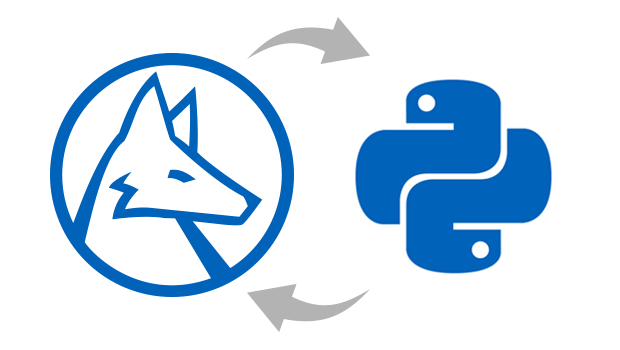“The Wolfram Client Library for Python is fully open source.”

## It’s Easy

The Wolfram Client Library makes it easy to integrate the large collection of Wolfram Language algorithms as well as the Wolfram Knowledgebase directly into any Python code that you already have. This saves you considerable time and effort when developing new code. In this post, we’ll first show you how to set up a connection from Python to the Wolfram Language. Next, we’ll explore a few methods and examples you can use to do a computation in the Wolfram Language and then call it for use in your Python session. For a complete introductory tutorial and full reference documentation, visit the documentation home page for the Wolfram Client Library for Python.

## Evaluate Locally…

Let’s start with a simple example, which computes the mean and standard deviation of one million numbers drawn from a normal distribution. This example shows how to call a Wolfram Language function from Python and compares the results from Python and the Wolfram Language to show that they are numerically close to one another.

### Statistical Data Analysis

First, to connect to the Wolfram Language, you need to create a new session with the Wolfram Engine:

```>>> from wolframclient.evaluation import WolframLanguageSession
>>> session = WolframLanguageSession()
```
&#10005

```from wolframclient.evaluation import WolframLanguageSession
session=WolframLanguageSession()```

To call Wolfram Language functions, you need to import the `wl` factory:

```>>> from wolframclient.language import wl
```
&#10005

`from wolframclient.language import wl`

Now you can evaluate any Wolfram Language code. Set the Python variable sample to a list of one million random numbers drawn from the normal distribution, with a mean of 0 and a standard deviation of 1:

```>>> sample = session.evaluate(wl.RandomVariate(
wl.NormalDistribution(0,1), 1e6))
```
&#10005

`sample = session.evaluate( wl.RandomVariate(wl.NormalDistribution(0,1), 1e6))`

You can take a look at the first five of them:

```>>> sample[:5]
[0.44767075774581,
0.9662810005828261,
-1.327910570542906,
-0.2383857558557122,
1.1826399551062043]
```
&#10005

`sample[:5]`

You can compute the mean value of this sample with the Wolfram Language. As expected, it is close to zero:

```>>> session.evaluate (wl.Mean(sample))
0.0013371607703851515
```
&#10005

`session.evaluate(wl.Mean(sample))`

You can also directly compute this in Python, to verify that you get a numerically similar result:

```>>> from statistics import mean
>>> mean(sample)
0.0013371607703851474
```
&#10005

```from statistics import mean
mean(sample)```

Similarly, you can compute the standard deviation of sample with the Wolfram Language:

```>>> session.evaluate(wl.StandardDeviation(sample))
1.0014296230797068
```
&#10005

`session.evaluate(wl.StandardDeviation(sample))`

Again run the following code in Python to verify that you get a similar result:

```>>> stdev(sample)
1.0014296230797068
```
&#10005

`stdev(sample)`

It’s good to see that these results are comparable. Now you know how to call some simple Wolfram Language functions from Python. Let’s continue with a more exciting example.

### Using the Wolfram Knowledgebase

Let’s take a look at a built-in Wolfram Language function that’s not readily available in Python, WolframAlpha:

```>>> moons = session.evaluate(wl.WolframAlpha('moons of Saturn', 'Result'))
```
&#10005

`moons = session.evaluate( wl.WolframAlpha('moons of Saturn', 'Result'))`

The WolframAlpha function is one of the high-level functions in the Wolfram Language that interacts with the Wolfram|Alpha servers via a web API. You can use this API directly from Python, but doing it by calling the WolframAlpha function is much more powerful and convenient because you can access all the data framework functions from the Wolfram Language directly. Let’s take a look at what the Python variable moons contains:

```>>> moons
EntityClass['PlanetaryMoon', 'SaturnMoon']
```
&#10005

`moons`

The output here is the Python representation of a Wolfram Language expression, which can be reused in any subsequent evaluation. For example, if you want to get the list of Saturn’s first four moons (by proximity) explicitly, you can do this:

```>>> session.evaluate(wl.EntityList(moons))[:4]
[Entity['PlanetaryMoon', 'S2009S1'],
Entity['PlanetaryMoon', 'Pan'],
Entity['PlanetaryMoon', 'Daphnis'],
Entity['PlanetaryMoon', 'Atlas']]
```
&#10005

`session.evaluate(wl.EntityList(moons))[:4]`

Or you can easily get the four largest moons of Saturn by mass with this small snippet of code:

```>>> bigmoons = session.evaluate(wl.EntityList(
wl.SortedEntityClass(moons, wl.Rule("Mass","Descending"),4)))
>>> bigmoons
[Entity['PlanetaryMoon', 'Titan'],
Entity['PlanetaryMoon', 'Rhea'],
Entity['PlanetaryMoon', 'Iapetus'],
Entity['PlanetaryMoon', 'Dione']]
```
&#10005

```bigmoons = session.evaluate(wl.EntityList(
wl.SortedEntityClass(moons, wl.Rule("Mass","Descending"),4)))
bigmoons```

And you can get a simple array of strings with the names of these moons like this:

```>>> session.evaluate(wl.Map(wl.Function(wl.Slot()("Name")), bigmoons))
['Titan', 'Rhea', 'Iapetus', 'Dione']
```
&#10005

`session.evaluate(wl.Map(wl.Function( wl.Slot()("Name")), bigmoons))`

This is all pretty cool. Let’s take a look at another example, using the Wolfram Language’s built-in image processing and machine learning functions.

### Image Processing and Machine Learning

First, let’s switch over to another mode to do evaluations directly in the Wolfram Language. So far you’ve used the `wl` factory to build up Wolfram Language expressions in Python. But you can also evaluate Python strings containing Wolfram Language code, and sometimes this is easier to read:

```>>> from wolframclient.language import wlexpr
```
&#10005

`from wolframclient.language import wlexpr`

For example, you can evaluate 1+1 in the Wolfram Language by sending it as a string:

```>>> session.evaluate('1+1')
2
```
&#10005

`session.evaluate('1+1')`

Using this method, you can write a small snippet of Wolfram Language code that takes an image and uses the built-in face-detection algorithm to find the location of a face in an image. Here, the image we’re using is the famous painting titled Girl with a Pearl Earring by the Dutch painter Johannes Vermeer (but it works on almost any image with recognizable faces). Because the Python terminal interface does not support the display of images, we’ll need use a Jupyter notebook instead, together with the Python Image Library (PIL) package, to help with displaying the result: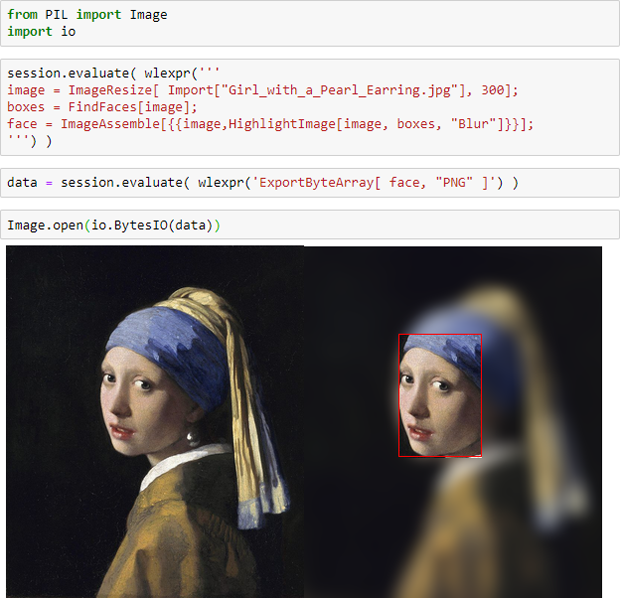✕ ```from PIL import Image import io session.evaluate( wlexpr(''' image = ImageResize[ Import["Girl_with_a_Pearl_Earring.jpg"], 300]; boxes = FindFaces[image]; face = ImageAssemble[{{image,HighlightImage[image, boxes, "Blur"]}}]; ''') ) data = session.evaluate( wlexpr('ExportByteArray[ face, "PNG" ]') ) Image.open(io.BytesIO)```

Quite easy and powerful. But what if you don’t have a local installation of the Wolfram Engine, and want to use the Wolfram Client Library for Python? You can still use the Wolfram Language directly in the Wolfram Cloud.

## To the Cloud

The Wolfram Cloud provides easy access to the Wolfram Language without needing to install it locally. The Wolfram Cloud provides various services, including a notebook web interface for Wolfram Language programming as well as the capability to deploy arbitrary Wolfram Language web APIs.

Here you’ll make use of the latter, deploying a Wolfram Language web API. This particular API accepts the names of two countries (country1 and country2), finds the capital city for each country and then computes the distance between them (in kilometers):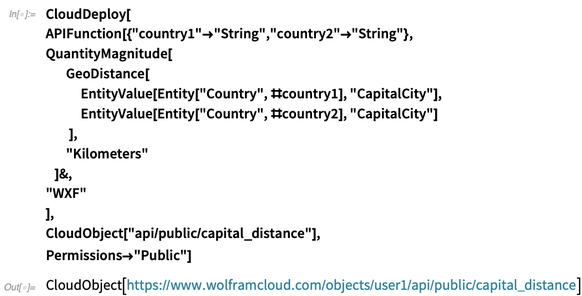✕ ```CloudDeploy[ APIFunction[{"country1"->"String","country2"->"String"}, QuantityMagnitude[ GeoDistance[ EntityValue[Entity["Country", #country1], "CapitalCity"], EntityValue[Entity["Country", #country2], "CapitalCity"] ], "Kilometers" ]&, "WXF" ], CloudObject["api/public/capital_distance"], Permissions->"Public"]```

After the deployment of this API, you can start a new Wolfram Language session, but this time you connect to the Wolfram Cloud instead of the local desktop engine:

```>>> from wolframclient.evaluation WolframCloudSession
>>> cloud = WolframCloudSession()
```
&#10005

```from wolframclient.evaluation WolframCloudSession
cloud = WolframCloudSession()```

To call the API, you have to provide the username (user1) and the API endpoint (api/public/capital_distance). With that information, you can call the cloud…

```>>> api = ('user1', 'api/public/capital_distance')
>>> result = cloud.call(api, {'country1': 'Netherlands',
'country2': 'Spain'})
```
&#10005

```api = ('user1', 'api/public/capital_distance')
result = cloud.call(api, {'country1': 'Netherlands', 'country2': 'Spain'})```

… and get the result:

```>>> result.get()
1481.4538329484521
```
&#10005

`result.get()`

Once again, easy and useful.

If you want to keep your deployed Wolfram Language API private, so that only you can use it, you can deploy the API with Permissions "Private". Then, to authenticate yourself to the private API, you can generate (in the Wolfram Language) a secured authentication key: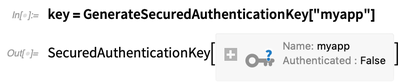✕ `key = GenerateSecuredAuthenticationKey["myapp"]`

Copy the outputs from these two inputs: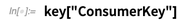✕ `key["ConsumerKey"]`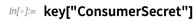✕ `key["ConsumerSecret"]`

Then paste them into your Python session:

```>>> from wolframclient.evaluation import SecuredAuthenticationKey
>>> sak = SecuredAuthenticationKey(
...    '<<paste-consumer-key-here>>',
...    '<<paste-consumer-secret-here>>')
```
&#10005

```from wolframclient.evaluation import SecuredAuthenticationKey
sak = SecuredAuthenticationKey(
...    '<>',
...    '<>')```

And finally, start a new authenticated cloud session:

```>>> cloud = WolframCloudSession(credentials=sak)
>>> cloud.start()
>>> cloud.authorized()
True
```
&#10005

```cloud = WolframCloudSession(credentials=sak)
cloud.start()
cloud.authorized()```

That’s it. At this point you (and only you) can use any Wolfram Language API that you have deployed privately.

## A Bit about the Underlying Serialization

To make everything very fast and efficient, the Wolfram Client Library for Python uses the open WXF format to exchange expressions between Python and the Wolfram Language. WXF is a binary format for faithfully serializing Wolfram Language expressions, in a form suitable for interchange with external programs. The library function export can serialize Python objects to string input form and WXF, and natively supports a set of built-in Python classes such as dict, list and strings:

```>>> from wolframclient.serializers import export
>>> export({
'list': [1,2,3],
'string': u'abc',
'etc': [0, None, -1.2]
})
b'<|"list" -> {1, 2, 3}, "string" -> "abc", "etc" -> {0, None, -1.2}|>'
```
&#10005

```from wolframclient.serializers import export
export({
'list': [1,2,3],
'string': u'abc',
'etc': [0, None, -1.2]
})
b'<|"list" -> {1, 2, 3}, "string" -> "abc", "etc" -> {0, None, -1.2}|>'```

WXF represents numeric arrays with packed data, allowing efficient support for NumPy arrays.

Create a new array of 255 unsigned 8-bit integers:

```>>> import numpy
>>> array=numpy.arange(255, dtype='uint8')
```
&#10005

```import numpy
array=numpy.arange(255, dtype='uint8')```

Serialize it to WXF bytes and compute the byte count:

```>>> wxf=export(array, target_format='wxf')
>>> len(wxf)
262
```
&#10005

```wxf=export(array, target_format='wxf')
len(wxf)```

NumPy arrays back many Python libraries. Therefore, an efficient and compact serialization helps in interfacing the Python ecosystem with the Wolfram Language. A direct consequence of supporting NumPy is that the serialization of PIL images is in general very efficient; most of the pixel data modes map to one of the numeric array types, specified by NumericArrayType. It’s also worth mentioning that pandas Series and DataFrame are supported natively. The library also provides an extensible mechanism for serializing arbitrary classes.

## Available Now

Install the latest version of the Wolfram Client Library for Python with pip: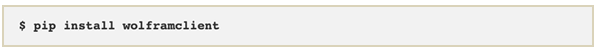✕ `\$ pip install wolframclient`

It requires Python 3.5.3 (or above) and Wolfram Language 11.3 (or above). Check out the full documentation on the Wolfram Client Library for Python. The entire source code is hosted in the WolframClientForPython repository on the Wolfram Research GitHub site. And if you see a way to improve it, you can help us make it better by contributing pull requests to this repository.

We’re very excited about this release and hope you find it useful. Let us know what you think in the comments section or on Wolfram Community, and we’ll do our best to respond to questions.